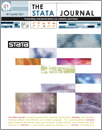Home  >>  Archives  >>  Volume 6 Number 1  >>  st0097

The Stata JournalVolume 6 Number 1: pp. 58-82

Subscribe to the Stata JournalGeneralized ordered logit/partial proportional odds models for ordinal dependent variables

 Richard Williams Department of Sociology University of Notre Dame Notre Dame, IN richard.a.williams.5@nd.edu
Abstract.   This article describes the gologit2 program for generalized ordered logit models. gologit2 is inspired by Vincent Fu’s gologit routine (Stata Technical Bulletin Reprints 8: 160–164) and is backward compatible with it but offers several additional powerful options. A major strength of gologit2 is that it can fit three special cases of the generalized model: the proportional odds/parallel-lines model, the partial proportional odds model, and the logistic regression model. Hence, gologit2 can fit models that are less restrictive than the parallel-lines models fitted by ologit (whose assumptions are often violated) but more parsimonious and interpretable than those fitted by a nonordinal method, such as multinomial logistic regression (i.e., mlogit). Other key advantages of gologit2 include support for linear constraints, survey data estimation, and the computation of estimated probabilities via the predict command.

View all articles by this author: Richard Williams

View all articles with these keywords: gologit2, gologit, logistic regression, ordinal regression, proportional odds, partial proportional odds, generalized ordered logit model, parallel-lines model